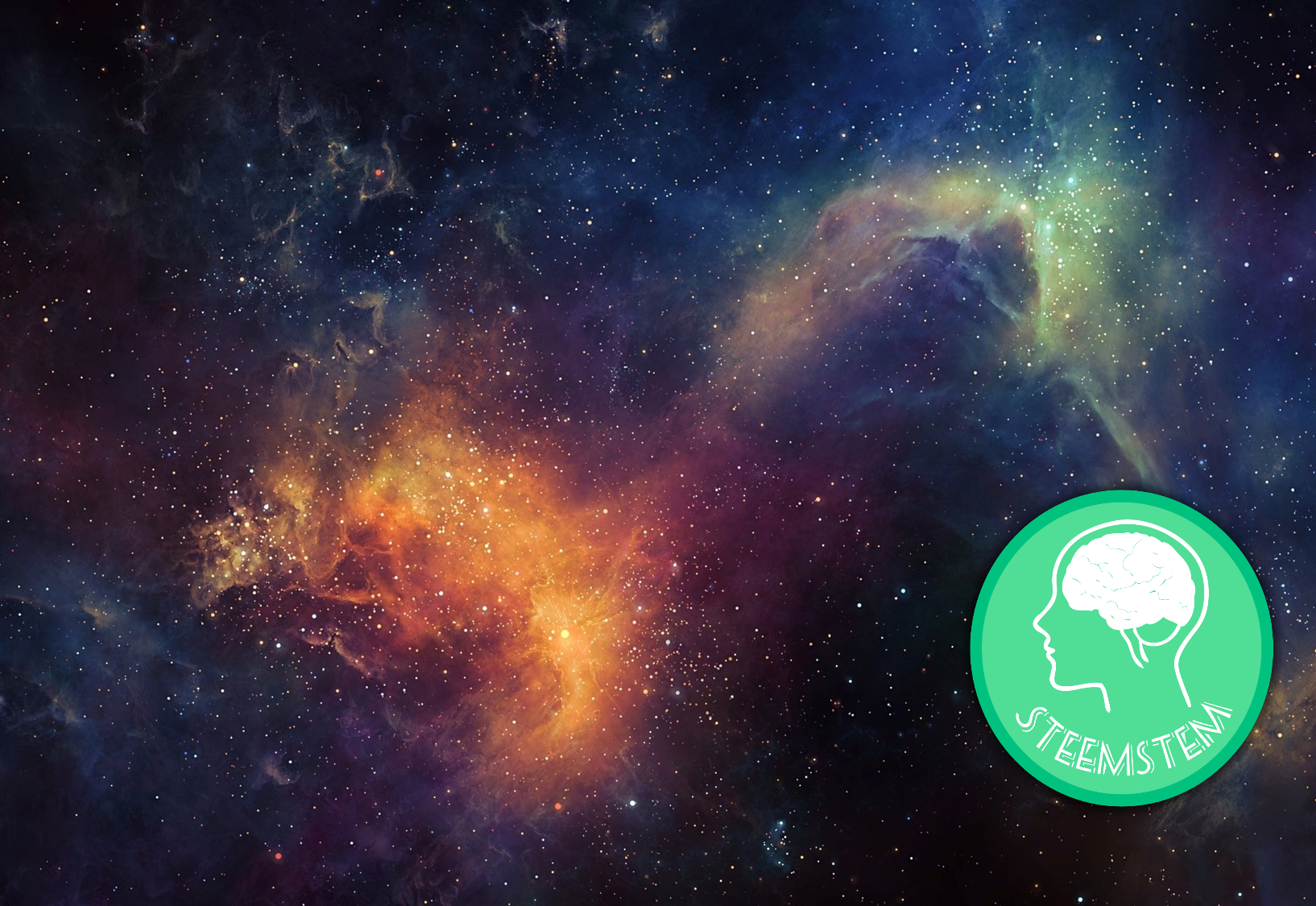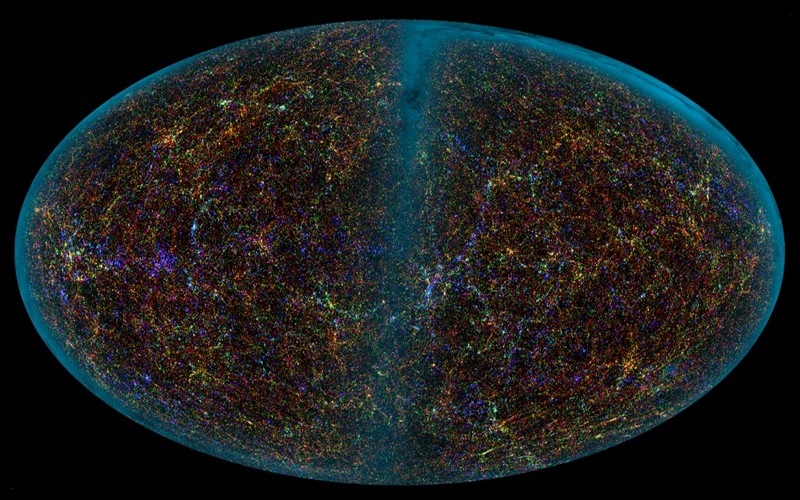# What Is The Shape Of The Universe?

in #steemstem4 years ago (edited)

The universe is the collection of all space and time. This practically includes everything that is existential: molecules, you, cities, planets, stars, galaxies, etc. So, the question is: is there a finite amount of space and time? The direct answer to this question is: maybe(?). Breaking the bad news first; no one knows the true shape of the universe. We simply do not have enough data to formulate a conclusion to our problem. However, we still have plenty to discuss considering we have theories supporting the potential for an infinite vs. a finite universe. Depending on the geometry of the universe this could result in either of the two.

Let’s cut straight to the point, a common question asked is: "Does the universe loop"? Assuming we never aged, moved at the speed of light and traveled in a straight line towards one direction is it possible that we could end up back where we were? Think of Pac-Man, where, if you go to the edge of the map you'd eventually loop back to the other side of the map. This is a fundamental question scientist are trying to answer in order to get a better understanding about the geometry of our universe.Media Source: http://eskipaper.com/free-desktop-wallpaper-5.html

Unfortunately, we still do not have enough data to conclude that we would “loop” around the universe. In order to check if looping is a possibility we have to measure the edge of our observable universe and find similarities in both ends. If the same galaxy can be found moving 86 billion light years west, and moving 86 billion light years east then we can make a relatively confident conclusion that our universe loops. Since we still do not see any identical formations of matter on either end we don’t know if it loops.

Arguably we know that space can be “bent” by mass; this is according to Einstein’s theory of general relativity. If we think of space as some sort of stretched out fabric and we place a ball somewhere on that fabric it will cause items on the fabric to “gravitate” towards it. This is because the fabric is “indented” or curved by the ball. Our real-time space works similarly. Massive bodies of matter cause gravity because matter is concentrated energy. This is where the application of E=mc^2 equation derives from.

Why do we care about this? Well, knowing this we can theorize the shape of the universe. We know 1 thing about the universe, and that is: it is constantly expanding. In fact, this is how we are able to derive the Big Bang Theory. The Big Bang Theory is the idea that the universe started from a single point of nearly infinite energy and “exploded” or expanded out into the universe we know today. Since the universe is still expanding, we assume the inverse relationship is that it can recede. This observable growth of the universe allows us to formulate ideas on what the potential shape of the universe could be!Media Source: https://cdn.zmescience.com/

The majority of individuals assume 3 geometric shapes of the universe: flat, spherical, and curved. More formally scientists say that the universe can have zero curvature, positive curvature or negative curvature. As stated above; general relativity forms a relation between mass and energy bending space. We can use this to formulate a parameter known as the density parameter which is defined to be the ratio of actual density to the critical density of the universe. Critical density is the value at which the universe is at a balance and expansion stops. Without getting too formal; just try to understand that the density parameter is the expected density of the universe over the critical density. By the laws of physics, we can use this to get a visual representation of the geometric shape of the universe.

Expanding on and simplifying the paragraph above: If the density parameter = 1 the universe is flat, if the density parameter is greater than 1 the universe is spherical, if the density parameter is less than 1 the universe has negative curvature (‘half-donut’ shape).

I’m not entirely sure what the majority of people believe the shape of the universe is, but growing up I was underneath the assumption that universe was ‘flat’. This flat universe is known as the Euclidean universe, which is modeled by Euclidean space. In this shape of the universe the question poses; is the universe infinite or finite? In the other shapes; positive and negative curvature, the universe could be finite, however you could argue infinity on the negative curvature.A Visual Representation of The Observable Universe

Now, as I learned more about geometry and our planet I wondered; what if the universe is round, more specifically spherical? It would make more ‘sense’ to me knowing that the universe is massively large and holds similar geometry to planets. In this theory; that the universe is spherical, is modeled by a the ‘n-sphere’ generalization; where in this case n = 3. This appears to be the most logical to me, however the evidence supporting this theory is relatively low. In the opposite direction with the negative curvature, is modeled by a region of hyperbolic space. This is something I did not even consider to be the shape of the universe until after conducing more research.

Remember these shapes are determined by the density parameter; which ultimately relies on how much density the universe holds. Since we can not actually measure the density of the universe; given that the “observable universe” isn’t the entire universe we don’t really know the shape of the universe. It’s all one big mystery that scientists are trying to solve. Either way, if you think the shape of the universe is mind blowing, wait till we learn more about the Euclidean universe and the outcomes of that being infinite!

Tl;DR: The universe is the collection of all space and time. This practically includes everything that is existential: molecules, you, cities, planets, stars, galaxies, etc. Considering that the universe is so vast; what is the shape of the universe? The answer: no one knows. We don’t even know how “big” the universe is. Scientists are constantly researching and discovering new things about the observable universe; keyword ‘observable’. We can only measure what we see, and the universe we see is a lot bigger than we can imagine.

Thanks to Einstein’s theory over general relativity scientist know that mass can ‘curve’ space. This idea can be seen where massive objects causes gravity (i.e planets, starts, etc.). Understanding this and using what’s known as the density parameter (the expected density of the universe over the critical density) we can assume 3 geometric shapes of the universe. These shapes are flat, spherical, and curved; more formally scientist like to say the universe has no curvature, positive curvature or negative curvature.

The density parameter helps us form a geometric representation of the universe: If the density parameter = 1 the universe is flat, if the density parameter is greater than 1 the universe is spherical, if the density parameter is less than 1 the universe has negative curvature (‘half-donut’ shape). Ultimately the density parameter relies on how much density the universe actually has, and due to us lacking the ability to measure the density of the universe we can not conclude the shape of the universe.

REFERENCES:

Note: A big thanks to @steemstem for providing fun and educational content relating to Science, Technology, Engineering and Math!Sort:

Mind bending :)

Congratulations @mindhacks, this post is the third most rewarded post (based on pending payouts) in the last 12 hours written by a Newbie account holder (accounts that hold between 0.01 and 0.1 Mega Vests). The total number of posts by newbie account holders during this period was 1999 and the total pending payments to posts in this category was \$441.32. To see the full list of highest paid posts across all accounts categories, click here.

If you do not wish to receive these messages in future, please reply stop to this comment.

STEEM 0.49
TRX 0.09
JST 0.062
BTC 49326.74
ETH 4179.15
BNB 566.65
SBD 5.99